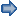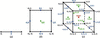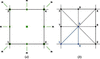## research papersFOUNDATIONSADVANCES
ISSN: 2053-2733
Volume 77| Part 2| March 2021| Pages 126-129

## Arithmetic proof of the multiplicity-weighted Euler characteristic for symmetrically arranged space-filling polyhedra

aFaculty of Mathematics and Computer Science, A. Mickiewicz University, Poznan, Poland, bMacromolecular Crystallography Laboratory, NCI, Argonne National Laboratory, Argonne, USA, cDepartment of Crystallography, Faculty of Chemistry, A. Mickiewicz University, Poznan, Poland, and dCenter for Biocrystallographic Research, Institute of Bioorganic Chemistry, Polish Academy of Sciences, Poznan, Poland
*Correspondence e-mail: mariuszj@amu.edu.pl

(Received 28 September 2020; accepted 12 December 2020; online 4 February 2021)

The puzzling observation that the famous Euler's formula for three-dimensional polyhedra VE + F = 2 or Euler characteristic χ = VE + FI = 1 (where V, E, F are the numbers of the bounding vertices, edges and faces, respectively, and I = 1 counts the single solid itself) when applied to space-filling solids, such as crystallographic asymmetric units or Dirichlet domains, are modified in such a way that they sum up to a value one unit smaller (i.e. to 1 or 0, respectively) is herewith given general validity. The proof provided in this paper for the modified Euler characteristic, χm = VmEm + FmIm = 0, is divided into two parts. First, it is demonstrated for translational lattices by using a simple argument based on parity groups of integer-indexed elements of the lattice. Next, Whitehead's theorem, about the invariance of the Euler characteristic, is used to extend the argument from the unit cell to its asymmetric unit components.

### 1. Introduction

The famous Euler characteristic that gives a simple relation between the numbers of geometrical elements of an isolated polytope in N-dimensional space can be expressed as follows:where ni is the number of i-dimensional cells (elements) building up the polytope. In the three-dimensional space this can be presented as an equation valid for any polyhedronwhere V, E, F, I are the numbers of vertices (0-dimensional elements), edges (one-dimensional elements), faces (two-dimensional elements) and the interior' or the polyhedron itself (three-dimensional element). We emphasize that each i-dimensional cell is considered always as a closed cell, i.e. with its boundary. Each individual polyhedron has only one interior' and Euler's formula is, therefore, often presented as VE + F = 2, as originally published by Euler (1758). The classical N-dimensional Euler characteristic of a polytope was derived by Schläfli (1901).

In a previous paper (Dauter & Jaskolski, 2020) in this journal we demonstrated, by analyzing standardized asymmetric units (ASUs) in all planar and space groups in the International Tables for Crystallography, Vol. A (Aroyo, 2016), as well as selected Dirichlet domains, that the polytopes in a lattice built from symmetrically arranged, space-filling polyhedra (or polygons in two dimensions) can be described by a modified Euler characteristic:where 1/m(i j) is the fraction which the individual element j of dimensionality i contributes to one selected polytope. In three-dimensional space this can also be expressed aswhere Vm, Em, Fm, Im represent total fractions of elements of different dimensionality ascribed to one polyhedron in the three-dimensional lattice. It is obvious that one polytope contains just one full interior' (representing in fact the complete solid), Im = 1, and that each face is always shared by two adjacent polyhedra so that Fm = F/2, but the values of Vm and Em depend on the particular arrangement of the polyhedra in the lattice. The convenient way of counting the contributions of individual elements to the modified Euler characteristic uses as their weights 1/m(ij) values that are inversely proportional to the number of adjacent polytopes m(ij) sharing a given element. Obviously, for three-dimensional polyhedra, m(31) = 1 (only one three-dimensional interior') and m(2j) = 2 (for any two-dimensional face).

Our considerations and musings on the modified Euler characteristic are not only of purely theoretical interest. For example, the modified Euler characteristic could find a very practical application in crystallography, as a kind of checksum' criterion in computer programs dealing with the ASU or Dirichlet domains.

Since when writing the first paper we were unable to construct a rigorous proof of the modified Euler's formula (or by extension of the modified Euler characteristic), we challenged our readers with this mathematical exercise. The response has been beyond expectations, with a number of contacts from fellow crystallographers received as soon as the electronic preprint became available. In particular, some colleagues recommended to us the classic textbook Regular Polytopes' by Coxeter (1948). On reading this book we realized that Coxeter's argument could be interpreted as a proof of the modified Euler characteristic, when applied to translational lattices. In particular, equation 4.82 from that book would be applicable to the three-dimensional case. A similar conclusion was reached by Dr Jean-Guillaume Eon (personal communication). An explanation of the rather complex line of Coxeter's thought is provided for interested readers in the supporting information. It is important to stress that, while Coxeter's proof is sufficient for translational lattices, or space filled with unit cells (hyper-parallelepipeds), it cannot be automatically extended to situations where the unit cell is subdivided into smaller polytopes, such as the ASU or Dirichlet domains, which in general do not populate the space by translations alone.

The goal of the present paper is to provide a complete proof of the modified Euler characteristic. We do it in two steps. First, we provide a simple proof applicable for translational lattices of any dimension N. This proof is based on the concept of parity frequencies of integer-indexed elements of the polytopes. This proof is alternative to the argument provided by Coxeter, but is based on a completely different arithmetic concept, is much simpler and is more general (applicable to any dimension N). In the second step, we use the theorem of Whitehead (1949) (cf. Hatcher, 2002) to extend the validity of the first step to polytopes that subdivide the unit cell in a symmetric fashion, but are not related by pure translations only. The second step completes the proof for ASUs and Dirichlet domains.

### 2. Arithmetic proof for translational lattices

A primitive lattice consists of points mutually related by basic translations along all existing dimensions of space. Their coordinates may be represented by sets of N integer numbers, where N is the dimensionality of the space. Such a lattice may also be treated as a periodic set of translationally arranged polytopes (parallelograms in two dimensions, parallelepipeds in three dimensions etc.). If the origin of the system of coordinates is selected at the center of one of the polytopes (unit cell) and the length of each edge (along each direction) is equal to two units of measure, then the centers of the constitutive elements (vertices, edges, faces etc.) of each cell will have coordinates expressed as sets of N integer numbers with different combinations of parity (even or odd, e/o) specific for each group of cell elements of particular dimensionality.

As illustrated in Fig. 1(a), in one-dimensional space, the lattice is a set of points (vertices) arranged periodically along a line. In one-dimensional space all vertices (0-dimensional elements) have odd coordinates (…−3; −1; 1; 3; 5; …), whereas the centers of all edges (one-dimensional elements) have even coordinates (…−2; 0; 2; 4; …).Figure 1 Regular polytopes with elements labeled by integer numbers and color (vertices, black; edges, blue; faces, green; interior', red): (a) one-dimensional case (line segment), (b) two-dimensional case (square), (c) three-dimensional case (cube).

In two-dimensional space, tiled by parallelograms [simplified in Fig. 1(b) as squares], all vertices have both coordinates odd (−1,1; 1,1; 1,3; 3,5; etc.), all edges have one coordinate even and one odd (0,1; 2,1; 3,2; etc.), and the centers of each face (two-dimensional elements) have both coordinates even (−2,−2; 0,0; 2,0; 2,4; etc.).

In three-dimensional space, tessellated by parallelepipeds [presented in Fig. 1(c) as cubes], all vertices have all three coordinates odd, all edge centers have one coordinate even and two odd, all face centers have one coordinate odd and two even, and all centers of the body (interiors') have all coordinates even.

The frequencies of groups of N integer numbers of different parities correspond to the frequencies of polytope elements of different dimensionalities in an N-dimensional lattice. Table 1presents these frequencies for infinite lattices and for a single isolated polytope. Since all the lattice-forming polytopes are identical, the lattice frequencies must be proportional to the concrete numbers of particular elements contributed by an individual polytope in the lattice, i.e. they can be used as contributions in the summation of the modified Euler characteristic. In particular, the frequency of the interior' element is always 1, underlying the fact that the Euler summation is for a single lattice-embedded cell. The values for a single isolated polytope in Table 1correspond to the standard Euler characteristic.

 Table 1Number of polytope elements, their parities and their coordinates, for the modified (infinite lattice) and standard (single polytope) Euler characteristic in spaces of different dimensions
Space elements Infinite lattice Single polytope
Number Parities Number Coordinates
One dimension, linear
Vertices 1 (o) 2 (−1),(1)
Edges 1 (e) 1 (0)
Two dimensions, planar
Vertices 1 (o,o) 4 (−1,−1); (−1,1); (1,−1); (1,1)
Edges 2 (e,o); (o,e) 4 (−1,0); (1,0); (0,−1), (0,1)
Faces 1 (e,e) 1 (0,0)
Three-dimensional space
Vertices 1 (o,o,o) 8 (−1,−1,−1) etc.
Edges 3 (e,o,o); (o,e,o); (o,o,e) 12 (0,−1,−1) etc.
Faces 3 (e,e,o); (e,o,e); (o,e,e) 6 (0,0,−1) etc.
Interior 1 (e,e,e) 1 (0,0,0)
N-dimensional space
0-dimensional elements (vertices) 1 (o)N 2N
One-dimensional elements (edges) N (e), (o)N−1 N×2N−1
i-dimensional elements ((N) || (i)) (e)i, (o)Ni ((N) || (i))×2Ni
N-dimensional element 1 (e)N 2N
Total elements 2N   3N
Euler characteristic†(e)x, (o)y means that there are x even numbers and y odd numbers in all permutations of their sequence.

For example, the modified Euler characteristic for one unit cell in the three-dimensional lattice built from paralellepipeds is χm = VmEm + FmIm = 1 − 3 + 3 − 1 = 0.

The arithmetic argument presented in this section provides in fact a proof of our main conjecture (vanishing Euler characteristic), valid for any dimension, but limited to the case of pure translations. We note that, because of the latter condition, this proof (as well as Coxeter's proof) does not encompass aperiodic tessellations of the space, such as Penrose tilings.

### 3. Extension to the asymmetric unit

Each polytope discussed in Section 2(parallelogram in two dimensions, parallelepiped in three dimensions etc.) can be divided into a number of identical, symmetrically arranged unique smaller parts (asymmetric units, ASUs) according to the space-group symmetry of the lattice. Such a procedure involves inclusion of additional elements (faces, edges etc.) into the unit cell and subdivision of the whole unit cell into smaller, identical, symmetry-equivalent polytopes.

The Euler characteristic of a polytope X is a quantity which is usually defined as the telescoping sumwhere ni is the number of i-cells of the polytope cellular decomposition (Steinitz & Rademacher, 1934). This definition clearly depends on the cellular structure of the polytope under consideration. However, one can express the Euler characteristic in the following way (cf. Hatcher, 2002, Theorem 2.44):where hi(X ) is the rank of the ith homology group of X. A fundamental theorem of topology, which goes back to the work of Whitehead (1949) about closure-finite weak (CW) complexes, is the claim that the (singular) homology groups of CW complexes (thus in particular of a polytope X with its cellular division) are invariant under particular decomposition of the space X into cells (Hatcher, 2002; p. 108).

To extend our discussion to the cases of the modified Euler characteristic of ASUs, we need to note the following general principle. For an n-fold covering mapof two CW complexes, i.e. a map with n preimages of a point, the Euler characteristic is preserved multiplicatively:(Spanier, 1982, p. 481, Theorem 3.1). The process of decomposition of a unit cell into identical copies of the ASU is necessarily associated with a covering map. It is natural then to consider the contributions of k-cells in the ASU as the contributions of k-cells of the cover divided by the number of copies of the ASU in the whole unit cell in the neighborhood of that k-cell. It is important to invoke the theorem of Whitehead (1949) mentioned above to free ourselves from the dependence on the choice of a particular cell decomposition.

This procedure is illustrated for the planar groups p4mm in Fig. 2. It is easier to visualize the symmetry relations in two dimensions than in three dimensions, but the conclusions are applicable to spaces of all dimensions.Figure 2 (a) The unit cell of the planar group p4mm (black square) with some of the symmetry elements shown in green. (b) Division of the unit cell into ASUs, with one ASU highlighted in blue.

A planar lattice built from square unit cells belongs to the p4mm symmetry group, as illustrated in Fig. 2. The ASU is a triangle withof the area of the whole unit cell. For the initial whole unit cell in Fig. 2(a) the modified Euler characteristic is χm = VmEm + Fm = 1 − 2 + 1 = 0, since all vertices are shared by 4 cells, Vm = 4 × ¼ = 1, all 4 edges are shared by 2 cells, Em = 4 × ½ = 2 and there is one unique face, Fm = 1, obviously not shared.

After division of the unit cell according to the p4mm symmetry, it consists of 8 smaller triangular faces (ASUs), shown in Fig. 2(b). Its 9 vertices and 16 edges are shared with neighboring unit cells in different degrees, so that the resulting modified Euler characteristic is χm = VmEm + Fm = 4 − 12 + 8 = 0. If only one triangular ASU is selected [1–5–6, marked in blue in Fig. 2(b)] as a representative polytope, it has the following Euler characteristic. Vertices 1 and 5 are shared by 8 neighbors each, and vertex 6 is shared by 4 neighbors, thus Vm =. All 3 edges are shared by 2 neighbors, thus Em = 3 × ½ = 1½. With 1 internal' face, Fm = 1, χm = VmEm + Fm = ½ − 1½ + 1 = 0. Since the whole unit cell is comprised of 8 triangular ASUs, the contributions Vm, Em, Fm of one individual ASU are 8 times smaller than for the whole unit cell, but the resulting value of χm is zero in both cases.

An illustration of the modified Euler characteristic calculations for crystallographic symmetry groups (including a case with translational symmetry elements) is presented in the supporting information for the examples of the two-dimensional space groups p4mm and pg, and for the three-dimensional space group P23.

### 4. Related literature

The following reference is cited in the supporting information: Cauchy (1813).

### Acknowledgements

We wish to thank all colleagues who showed interest in our work and suggested ways of attacking the proof.

### References

This is an open-access article distributed under the terms of the Creative Commons Attribution (CC-BY) Licence, which permits unrestricted use, distribution, and reproduction in any medium, provided the original authors and source are cited.FOUNDATIONSADVANCES
ISSN: 2053-2733
Volume 77| Part 2| March 2021| Pages 126-129